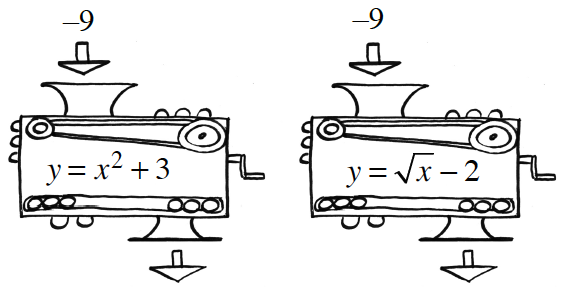### Home > INT1 > Chapter 1 > Lesson 1.1.2 > Problem1-24

1-24.FUNCTION MACHINES

1. If an input of $–9$ is put into each of the machines at right, what is each output?

Substitute $−9$ for $x$ in each of the equations in both the machines, then solve the equations for $y$.

2. Eric wants to get an output of $0$. Can he do this with each machine? If so, how? If not, why not?

• What numbers could Eric substitute in the equations to get $0$? Is it possible to get this answer from both machines?

Function A: No solution. Do you know why?